# 802.11be Transmitter Measurements

This example shows how to generate an IEEE® 802.11be™ single-user waveform with 4096-point quadrature amplitude modulation (4096-QAM) and a channel bandwidth of 320 MHz. The example demonstrates how to measure transmitter modulation accuracy, spectral mask, and spectral flatness.

### Introduction

This example shows how to parameterize and generate an IEEE 802.11be extremely high throughput (EHT) single-user waveform specified in P802.11be Draft 1.0 . This example performs transmitter modulation accuracy, required spectral mask, and required spectral flatness measurements on the waveform for the configuration specified in Section 36.3.19 of .

The example generates 20 single-user EHT packets with a 320 MHz channel bandwidth and a 10 microsecond gaps between packets. Each packet contains random data and uses 4096-QAM. The example oversamples the waveform using a larger IFFT than required for the nominal baseband rate and does not perform spectral filtering. The example uses a high-power amplifier (HPA) model, which introduces in-band distortion and spectral regrowth. The example performs spectral emission mask measurement on the upsampled waveform after the HPA modeling. The example decodes the EHT data field and measures the error vector magnitude (EVM) to determine the modulation accuracy after downsampling the waveform to baseband sampling rate. Additionally, the example measures the spectral flatness of the recovered waveform. This diagram shows the workflow contained in the example.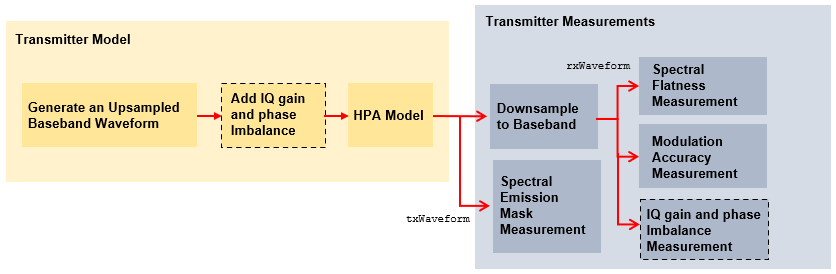### Simulation Setup

Configure the example to `generate` 20 single-user EHT packets with a 10 microsecond idle period between each packet.

```numPackets =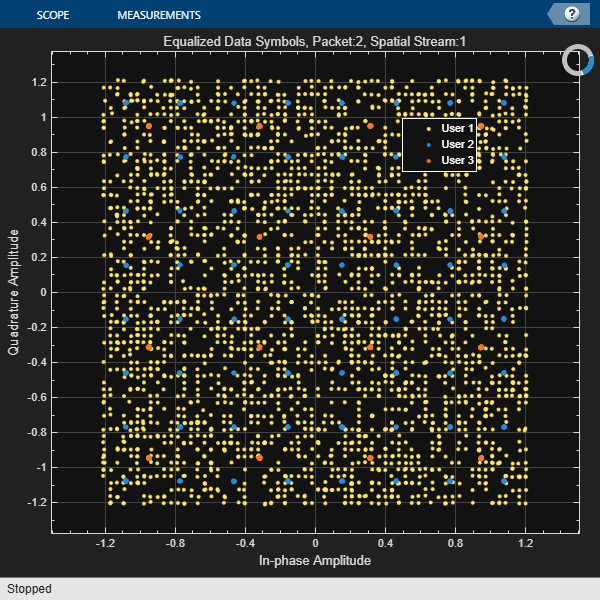20; idleTime =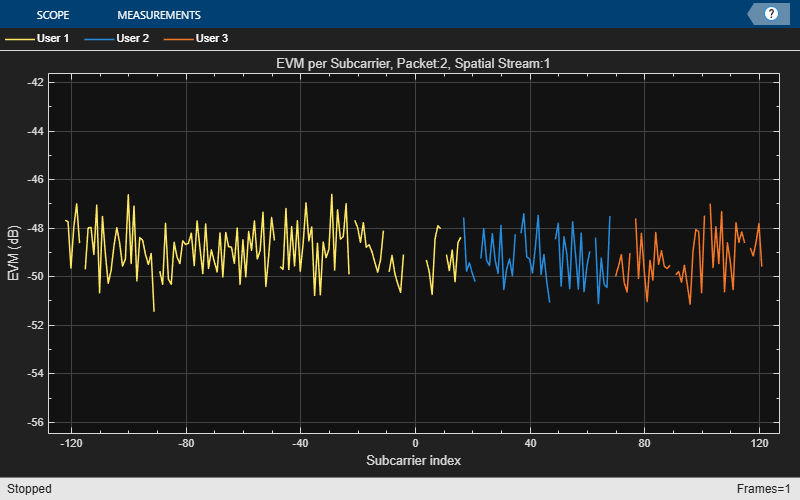10; % In microseconds```

### 802.11be Waveform Configuration and Generation

The physical layer of 802.11be, defined in P802.11be Draft 1.0 , builds upon 802.11ax  with these added capabilities and modifications.

1. Increased maximum channel bandwidth of 320 MHz

2. 4096-QAM in MCS index options 12 and 13

3. Assignment of multiple resource unit (MRU) to a single station (STA), with optional puncturing of single-user packets

4. Increased maximum number of spatial streams for uplink MU-MIMO (16 spatial streams across up to 8 users)

5. Modified tone plan for 80 and 160 MHz bandwidths

6. Larger generator polynomial for Data field scrambler and descrambler

This example models these key 802.11be features.

1. 320 MHz channel bandwidth

2. 4096-QAM modulation

3. Modified tone plan for 80 and 160 MHz bandwidths

4. Larger generator polynomial for Data field scrambler and descrambler

The draft standard defines the EHT multi-user (EHT MU) format for the transmission of both single-user and multi-user packets. This example only supports generation of single-user packets. Use the EHT MU configuration object, `ehtMUConfig`, to configure transmission properties of an EHT MU packet. This example uses random bits to generate the U-SIG and EHT-SIG signaling fields. You can specify the random seed or field bit values by modifying the relevant properties of the `ehtMUConfig` object. The value of the U-SIG and EHT-SIG bits affects the peak-to-average-power ratio (PAPR) of the packet. Because this example does not use space-time block coding, it can measure the modulation accuracy per spatial stream.

```chanBW =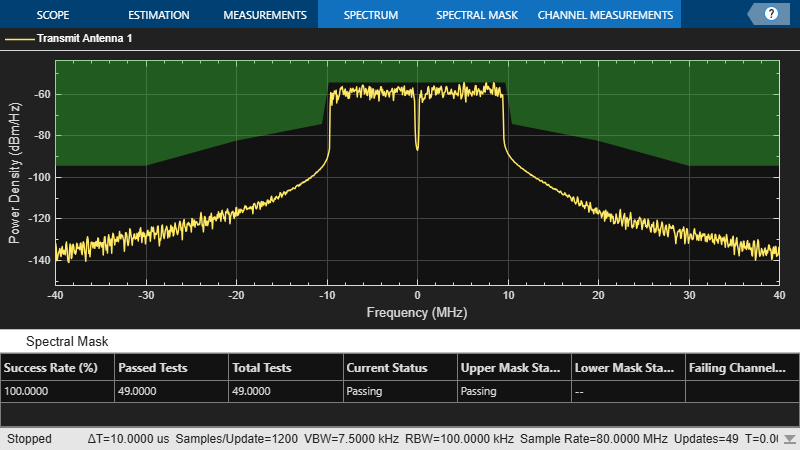'CBW320'; % Channel bandwidth mcs =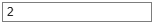12; % Modulation and coding scheme numTx =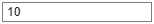1; % Number of transmit antennas apepLength =8000; % A-MPDU length pre-EOF padding in bytes savedState = rng(0); % Set random state cfgEHT = ehtMUConfig(chanBW); % EHT MU configuration object ```
```cfgEHT.NumTransmitAntennas = numTx; cfgEHT.User{1}.MCS = mcs; cfgEHT.User{1}.NumSpaceTimeStreams = numTx; cfgEHT.User{1}.APEPLength = apepLength; cfgEHT.RU{1}.SpatialMapping = 'Direct';```

To model the effect of a HPA on the waveform and view the out-of-band spectral emissions, the waveform must be oversampled. This example generates the waveform using a larger IFFT than required for the nominal baseband rate, resulting in an oversampled waveform. The example does not perform spectral filtering.

`osf =4; % Oversampling factor`

Create random bits for all packets.

```psduLength = cfgEHT.getPSDULength*8; % PSDU length in bits per packet data = randi([0 1],psduLength*numPackets,1);```

Generate the EHT MU waveform for the specified bits and configuration by using the `ehtWaveformGenerator` function, specifying the desired oversampling factor, number of packets, and idle time between each packet.

`txWaveform = ehtWaveformGenerator(data,cfgEHT,'NumPackets',numPackets,'IdleTime',idleTime*1e-6,'OversamplingFactor',osf);`

Get the baseband sampling rate of the waveform

```fs = wlanSampleRate(chanBW); disp(['Baseband sampling rate: ' num2str(fs/1e6) ' Msps']);```

Prepend zeros to the waveform to allow for early timing synchronization.

`txWaveform = [zeros(round(idleTime*1e-6*fs),numTx); txWaveform];`

#### HPA Modeling

The HPA introduces nonlinear behavior in the form of in-band distortion and spectral regrowth. This example simulates the power amplifiers by using the Rapp model , which introduces AM/AM distortion.

Model the amplifier by using the `dsp.FIRInterpolator` object, and configure reduced distortion by specifying a back-off, `hpaBackoff`, such that the amplifier operates below its saturation point. You can increase the backoff to reduce EVM for higher MCS values.

```pSaturation = 25; % Saturation power of a power amplifier in dBm hpaBackoff = 16; % Power amplifier backoff in dB nonLinearity = comm.MemorylessNonlinearity; nonLinearity.Method = 'Rapp model'; nonLinearity.Smoothness = 3; % p parameter nonLinearity.LinearGain = -hpaBackoff; nonLinearity.OutputSaturationLevel = db2mag(pSaturation-30); txWaveform = nonLinearity(txWaveform);```

#### Thermal Noise

Add thermal noise to each transmit antenna by using the `comm.ThermalNoise` object with a noise figure of 6 dB .

```thNoise = comm.ThermalNoise('NoiseMethod','Noise Figure','SampleRate',fs*osf,'NoiseFigure',6); for i = 1:cfgEHT.NumTransmitAntennas txWaveform(:,i) = thNoise(txWaveform(:,i)); end```

### Modulation Accuracy (EVM) and Spectral Flatness Measurements

#### Downsampling and Filtering

Resample the oversampled waveform down to baseband for physical layer processing and EVM and spectral flatness measurements, applying a low-pass anti-aliasing filter before downsampling. The impact of the low-pass filter is visible in the spectral flatness measurement. The anti-aliasing filter is designed so that all active subcarriers are within the filter passband.

Design resampling filter.

```aStop = 40; % Stopband attenuation ofdmInfo = ehtOFDMInfo('EHT-Data',cfgEHT); % OFDM parameters SCS = fs/ofdmInfo.FFTLength; % Subcarrier spacing txbw = max(abs(ofdmInfo.ActiveFrequencyIndices))*2*SCS; % Occupied bandwidth [L,M] = rat(osf); maxLM = max([L M]); R = (fs-txbw)/fs; TW = 2*R/maxLM; % Transition width b = designMultirateFIR(L,M,TW,aStop);```

Resample the waveform to baseband.

```firinterp = dsp.FIRRateConverter(M,L,b); rxWaveform = firinterp(txWaveform);```

This section detects, synchronizes, and extracts each packet in `rxWaveform`, then measures the EVM and spectral flatness. For each packet, the example performs these steps.

• Detect the start of the packet

• Extract the legacy fields

• Estimate and correct coarse carrier frequency offset (CFO)

• Perform fine symbol timing estimate by using the frequency-corrected legacy fields

• Extract the packet from the waveform by using the fine symbol timing offset

• Correct the extracted packet with the coarse CFO estimate

• Extract the L-LTF, then estimate and correct the fine CFO

• Extract the EHT-LTF and perform channel estimation for each of the transmit streams

• Measure the spectral flatness by using the channel estimate

• Extract and OFDM demodulate the EHT Data field

• Perform noise estimation by using the demodulated data field pilots and single-stream channel estimate at pilot subcarriers

• Phase-correct and equalize the EHT Data field by using the channel and noise estimates

• For each data-carrying subcarrier in each spatial stream, find the closest constellation point and measure the EVM

• Recover the PSDU by decoding the equalized symbols

This diagram shows the processing chain.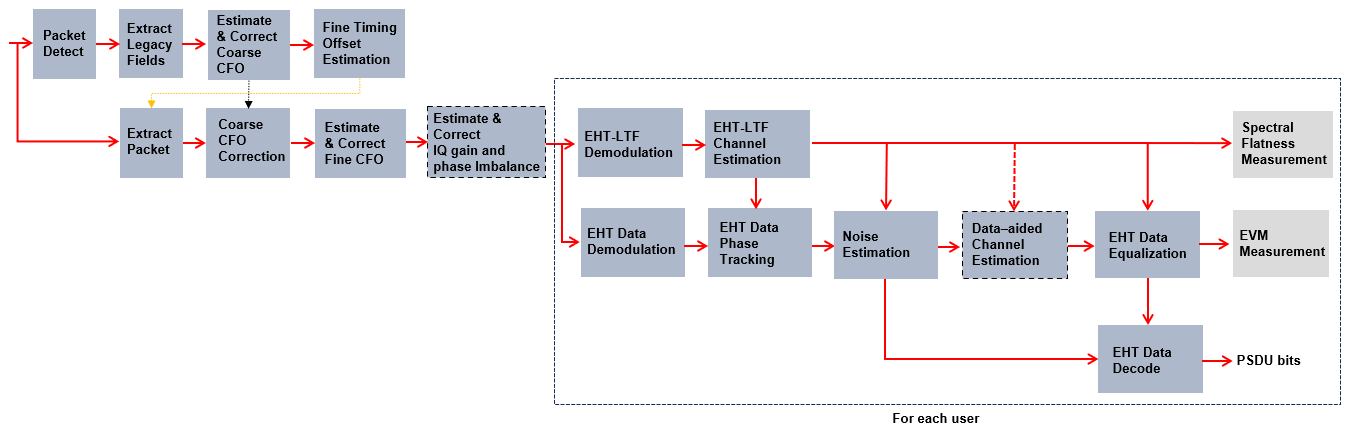This example makes two different EVM measurements.

1. RMS EVM per packet, which comprises averaging the EVM over subcarriers, OFDM symbols, and spatial streams.

2. RMS EVM per subcarrier per spatial stream for a packet. Because this configuration maps spatial streams directly to antennas, this measurement can help detect frequency-dependent impairments, which may affect individual RF chains differently. This measurement averages the EVM over OFDM symbols only.

Setup EVM measurements.

`[EVMPerPkt,EVMPerSC] = evmSetup(cfgEHT);`

Get indices for accessing each field within the time-domain packet.

`ind = ehtFieldIndices(cfgEHT);`

Define the minimum detectable length of data, in samples.

`minPktLen = double(ind.LSTF(2)-ind.LSTF(1))+1;`

Detect and process packets within the received waveform by using a `while` loop, which performs these steps.

1. Detect a packet by indexing into `rxWaveform` with the sample offset, `searchOffset`

2. Detect and process the first packet within `rxWaveform`

3. Detect and process the next packet by incrementing the sample index offset

4. Repeat until no further packets are detected

```rxWaveformLength = size(rxWaveform,1); pktLength = double(ind.EHTData(2)); rmsEVM = zeros(numPackets,1); pktOffsetStore = zeros(numPackets,1); rng(savedState); % Restore random state pktNum = 0; searchOffset = 0; % Start at first sample (no offset) while (searchOffset+minPktLen)<=rxWaveformLength % Detect packet and determine coarse packet offset pktOffset = wlanPacketDetect(rxWaveform,cfgEHT.ChannelBandwidth,searchOffset); % Packet offset from start of the waveform pktOffset = searchOffset+pktOffset; % Skip packet if L-STF is empty if isempty(pktOffset) || (pktOffset<0) || ... ((pktOffset+ind.LSIG(2))>rxWaveformLength) break; end % Extract L-STF and perform coarse frequency offset correction nonht = rxWaveform(pktOffset+(ind.LSTF(1):ind.LSIG(2)),:); coarsefreqOff = wlanCoarseCFOEstimate(nonht,cfgEHT.ChannelBandwidth); nonht = helperFrequencyOffset(nonht,fs,-coarsefreqOff); % Extract the legacy fields and determine fine packet offset lltfOffset = wlanSymbolTimingEstimate(nonht,cfgEHT.ChannelBandwidth); pktOffset = pktOffset+lltfOffset; % Determine packet offset % If offset is outwith bounds of the waveform, then skip samples and % continue searching within remainder of the waveform if (pktOffset<0) || ((pktOffset+pktLength)>rxWaveformLength) searchOffset = pktOffset+double(ind.LSTF(2))+1; continue; end % Timing synchronization complete; extract the detected packet rxPacket = rxWaveform(pktOffset+(1:pktLength),:); pktNum = pktNum+1; disp([' Packet ' num2str(pktNum) ' at index: ' num2str(pktOffset+1)]); % Apply coarse frequency correction to the extracted packet rxPacket = helperFrequencyOffset(rxPacket,fs,-coarsefreqOff); % Perform fine frequency offset correction on the extracted packet lltf = rxPacket(ind.LLTF(1):ind.LLTF(2),:); % Extract L-LTF fineFreqOff = wlanFineCFOEstimate(lltf,cfgEHT.ChannelBandwidth); rxPacket = helperFrequencyOffset(rxPacket,fs,-fineFreqOff); % Extract EHT-LTF samples, demodulate, and perform channel estimation ehtLTF = rxPacket(ind.EHTLTF(1):ind.EHTLTF(2),:); ehtLTFDemod = ehtDemodulate(ehtLTF,'EHT-LTF',cfgEHT); % Channel estimate [chanEst,pilotEst] = ehtLTFChannelEstimate(ehtLTFDemod,cfgEHT); % Spectral flatness measurement ehtTxSpectralFlatnessMeasurement(chanEst,cfgEHT,pktNum); % Data demodulate rxData = rxPacket(ind.EHTData(1):ind.EHTData(2),:); demodSym = ehtDemodulate(rxData,'EHT-Data',cfgEHT); % Pilot phase tracking demodSym = ehtCommonPhaseErrorTracking(demodSym,chanEst,cfgEHT); % Estimate noise power in EHT fields nVarEst = ehtNoiseEstimate(demodSym(ofdmInfo.PilotIndices,:,:),pilotEst,cfgEHT); % Extract data subcarriers from demodulated symbols and channel % estimate demodDataSym = demodSym(ofdmInfo.DataIndices,:,:); chanEstData = chanEst(ofdmInfo.DataIndices,:,:); % Equalization and STBC combining [eqSym,csi] = ehtEqualizeCombine(demodDataSym,chanEstData,nVarEst,cfgEHT); % Compute RMS EVM over all spatial streams for a packet rmsEVM(pktNum) = EVMPerPkt(eqSym); fprintf(' RMS EVM: %2.2f%%, %2.2fdB\n',rmsEVM(pktNum),20*log10(rmsEVM(pktNum)/100)); % Compute RMS EVM per subcarrier and spatial stream for the packet evmPerSC = EVMPerSC(eqSym); % Nst-by-1-by-Nss % Plot RMS EVM per subcarrier and equalized constellation ehtTxEVMConstellationPlots(eqSym,evmPerSC,cfgEHT,pktNum); % Recover data field bits rxPSDU = ehtDataBitRecover(eqSym,nVarEst,csi,cfgEHT); if isequal(rxPSDU,data((1:psduLength)+(pktNum-1)*psduLength)) fprintf(' Decode success\n'); else fprintf(' Decode failure\n'); end % Store the offset of each packet within the waveform pktOffsetStore(pktNum) = pktOffset; % Increment waveform offset and search remaining waveform for a packet searchOffset = pktOffset+pktLength+minPktLen; end if pktNum>0 fprintf('Average EVM for %d packets: %2.2f%%, %2.2fdB\n', ... pktNum,mean(rmsEVM(1:pktNum)),20*log10(mean(rmsEVM(1:pktNum))/100)); else disp('No complete packet detected'); end```

This section measures the spectral mask of the filtered and impaired waveform after HPA modeling. The transmitter spectral mask test  uses a time-gated spectral measurement of the EHT Data field. The example extracts the EHT Data field of each packet from the oversampled waveform by using the start indices of each packet within the baseband waveform. Any delay introduced in the baseband processing chain used to determine the packet indices must be accounted for when gating the EHT data field within `txWaveform`. Concatenate the extracted EHT Data fields in preparation for measurement.

```startIdx = osf*(ind.EHTData(1)-1)+1; % Upsampled start of EHT Data endIdx = osf*ind.EHTData(2); % Upsampled end of EHT Data delay = grpdelay(firinterp,1); % Group delay of downsampling filter idx = zeros(endIdx-startIdx+1,pktNum); for i = 1:pktNum % Start of packet in txWaveform pktOffset = round(osf*pktOffsetStore(i))-delay; % Indices of EHT-Data in txWaveform idx(:,i) = (pktOffset+(startIdx:endIdx)); end gatedEHTTData = txWaveform(idx(:),:); ```

The 802.11be standard specifies the spectral mask relative to the peak power spectral density. This generated plot overlays the required mask with the measured PSD.

```if pktNum>0 ehtSpectralMaskTest(gatedEHTTData,fs,osf); end```

### Conclusion and Further Exploration

This example measures and plots:

• Spectral flatness

• RMS EVM per subcarrier

• Equalized constellation

The HPA model introduces significant in-band distortion and spectral regrowth, which is visible in the EVM results, noisy constellation, and out-of-band emissions in the spectral mask plot. Try increasing the HPA backoff and observe the improved EVM, constellation, and lower out-of-band emissions. The downsampling (to bring the waveform to baseband for processing) stage includes filtering. The filter response affects the spectral flatness measurement. The ripple in the spectral flatness measurement is due to downsampling to baseband. Try using a different filter or changing the stop-band attenuation and observe the impact on the spectral flatness.

### References

1. IEEE P802.11be™/D1.0 Draft Standard for Information technology — Telecommunications and information exchange between systems Local and metropolitan area networks — Specific requirements - Part 11: Wireless LAN Medium Access Control (MAC) and Physical Layer (PHY) Specifications - Amendment 8: Enhancements for extremely high throughput (EHT).

2. IEEE P802.11ax™/D4.1 Draft Standard for Information technology - Telecommunications and information exchange between systems - Local and metropolitan area networks - Specific requirements - Part 11: Wireless LAN Medium Access Control (MAC) and Physical Layer (PHY) Specifications - Amendment 1: Enhancements for High Efficiency WLAN.

3. Loc and Cheong. IEEE P802.11 Wireless LANs. TGac Functional Requirements and Evaluation Methodology Rev. 16. 2011-01-19.

4. Perahia, E., and R. Stacey. Next Generation Wireless LANs: 802.11n and 802.11ac. 2nd Edition. United Kingdom: Cambridge University Press, 2013.

5. Archambault, Jerry, and Shravan Surineni. "IEEE 802.11 spectral measurements using vector signal analyzers." RF Design 27.6 (2004): 38-49.

## SupportGet trial now